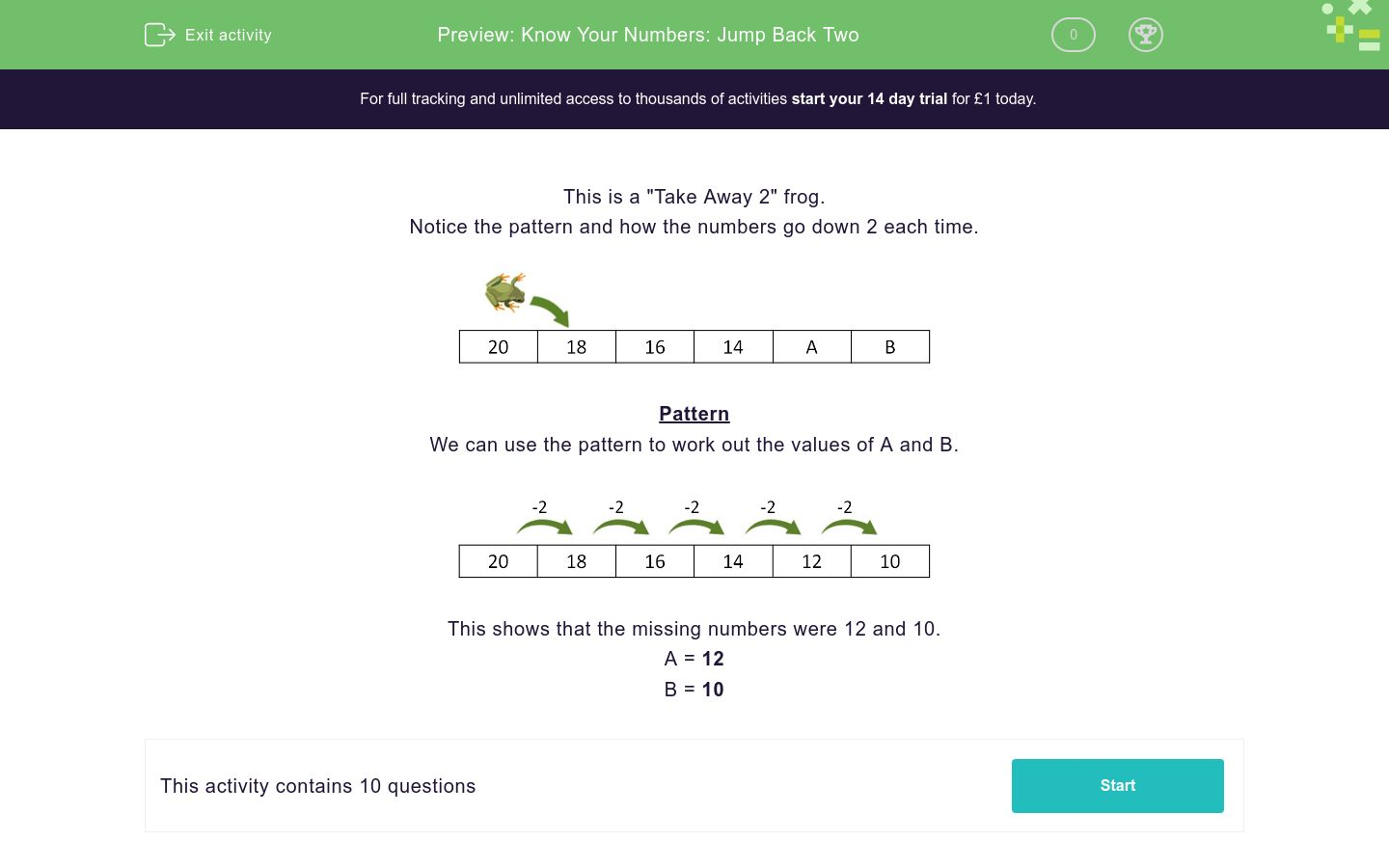# Know Your Numbers: Jump Back Two

In this worksheet, students find the missing numbers in a backward sequence that reduces each time by two.Key stage:  KS 1

Curriculum topic:   Number: Number and Place Value

Curriculum subtopic:   Count in Steps (2, 3, 5 and 10)

Difficulty level:### QUESTION 1 of 10

This is a "Take Away 2" frog.

Notice the pattern and how the numbers go down 2 each time.Pattern

We can use the pattern to work out the values of A and B.This shows that the missing numbers were 12 and 10.

A = 12

B = 10

This is a "Take Away 2" frog.

Work out the values of A and B.26 24 22 20 A B
 A B 13 18 12 16

This is a "Take Away 2" frog.

Work out the values of A and B.27 25 23 21 A B

 A B 16 19 18 17

This is a "Take Away 2" frog.

Work out the values of A and B.30 28 26 24 A B
 A B 20 25 22 26

This is a "Take Away 2" frog.

Work out the values of A and B.20 18 16 14 A B
 A B 13 10 12 16

This is a "Take Away 2" frog.

Work out the values of A and B.23 21 19 17 A B
 A B 13 15 12 16

This is a "Take Away 2" frog.

Work out the values of A and B.24 22 20 18 A B
 A B 13 14 12 16

This is a "Take Away 2" frog.

Work out the values of A and B.25 23 21 19 A B

 A B 13 17 12 15

This is a "Take Away 2" frog.

Work out the values of A and B.29 27 25 23 A B
 A B 20 21 18 19

This is a "Take Away 2" frog.

Work out the values of A and B.16 14 12 A 8 B
 A B 10 8 2 6

This is a "Take Away 2" frog.

Work out the values of A and B.26 24 A 20 18 B

 A B 16 24 22 26
• Question 1

This is a "Take Away 2" frog.

Work out the values of A and B.26 24 22 20 A B
 A B 13 18 12 16
• Question 2

This is a "Take Away 2" frog.

Work out the values of A and B.27 25 23 21 A B

 A B 16 19 18 17
• Question 3

This is a "Take Away 2" frog.

Work out the values of A and B.30 28 26 24 A B
 A B 20 25 22 26
• Question 4

This is a "Take Away 2" frog.

Work out the values of A and B.20 18 16 14 A B
 A B 13 10 12 16
• Question 5

This is a "Take Away 2" frog.

Work out the values of A and B.23 21 19 17 A B
 A B 13 15 12 16
• Question 6

This is a "Take Away 2" frog.

Work out the values of A and B.24 22 20 18 A B
 A B 13 14 12 16
• Question 7

This is a "Take Away 2" frog.

Work out the values of A and B.25 23 21 19 A B

 A B 13 17 12 15
• Question 8

This is a "Take Away 2" frog.

Work out the values of A and B.29 27 25 23 A B
 A B 20 21 18 19
• Question 9

This is a "Take Away 2" frog.

Work out the values of A and B.16 14 12 A 8 B
 A B 10 8 2 6
• Question 10

This is a "Take Away 2" frog.

Work out the values of A and B.26 24 A 20 18 B

 A B 16 24 22 26
---- OR ----

Sign up for a £1 trial so you can track and measure your child's progress on this activity.

### What is EdPlace?

We're your National Curriculum aligned online education content provider helping each child succeed in English, maths and science from year 1 to GCSE. With an EdPlace account you’ll be able to track and measure progress, helping each child achieve their best. We build confidence and attainment by personalising each child’s learning at a level that suits them.

Get started